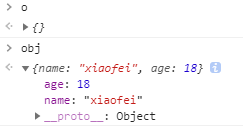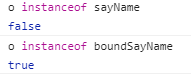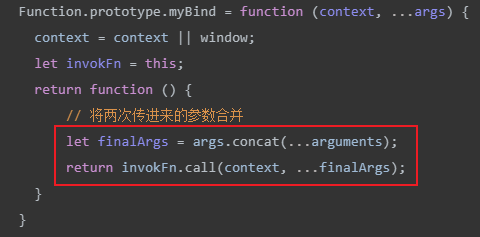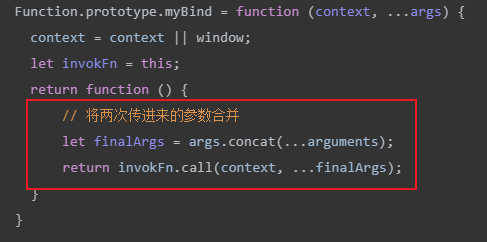# JS-bind方法的两种实现

charlesc

0收藏

1. 简单版

1.1 实现

(备注：简单版不支持使用new调用新创建的构造函数)

``````Function.prototype.myBind = function (context, ...args) {
context = context || window;
let invokFn = this;
return function () {
// 将两次传进来的参数合并
let finalArgs = args.concat(...arguments);
return invokFn.call(context, ...finalArgs);
}
}``````

``````let obj = {
name: "xiaofei"
}
function sayName(age, sex){
console.log(this.name, age+"岁", sex);
}
let boundSayName = sayName.myBind(obj, 18);
boundSayName("男");    // xiaofei 18岁 男``````

1.2 问题

``````let obj = {
name: "xiaofei"
}
function sayName(age){
console.log(this.name);    / **（1）** /
this.age = age;
}
let boundSayName = sayName.myBind(obj, 18);
let o = new boundSayName();    // xiaofei``````2. 升级版

2.1 问题的本质

``````function myNew(fn) {
let objTemp = {};
objTemp.__proto__ = fn.prototype;
let args = [].slice.call(arguments, 1);
let result = fn.call(objTemp, ...args);    /*（2）*/
return (typeof result === 'object' && result != null) ? result : objTemp;
}````````````Function.prototype.myBind = function (context, ...args) {
context = context || window;
let invokFn = this;
return function () {
// 将两次传进来的参数合并
let finalArgs = args.concat(...arguments);
return invokFn.call(context, ...finalArgs);
}
}

let obj = {
name: "xiaofei"
}
function sayName(age){
console.log(this.name);    / **（1）** /
this.age = age;
}
let boundSayName = sayName.myBind(obj, 18);
let o = new boundSayName();    // xiaofei

console.log(o);    // {}
console.log(obj);    // {name: "xiaofei", age: 18}
o instanceof sayName; // false
o instanceof boundSayName;    // true``````

age属性在o实例上，obj对象上没有age属性；
o既是sayName的实例，也是boundSayName的实例，即：o instanceof sayName 和 o instanceof boundSayName都返回true。

（2.2.1） 我们先来思考第一个问题。• 当boundFn的prototype出现在this对象的原型链中，说明此时是new调用的，此时call中传入this对象，也就是objTemp，也就是最终生成的实例；
• 如果不是，说明此时是普通执行（此时this指向window，window instanceof boundFn 显然返回false），call中就传入context对象，也就是obj对象。
代码如下：
``````Function.prototype.myBind = function (context, ...args) {
context = context || window;
let invokFn = this;
let boundFn = function () {
let finalArgs = args.concat(...arguments);
// 看这里！！就多了这里一行代码！！
return invokFn.call((this instanceof boundFn) ? this : context, ...finalArgs);
}
return boundFn;
}​``````

（2.2.2） 下面来看第二个问题：新生成的实例不是sayName的实例。

``````Function.prototype.myBind = function (context, ...args) {
context = context || window;
let invokFn = this;
let helperFn = function(){};    // 借助这个辅助函数将invokFn的prototype混入boundFn的原型链中.
helperFn.prototype = invokFn.prototype;
let boundFn = function () {
// 将两次传进来的参数合并
console.log(this instanceof invokFn);
let finalArgs = args.concat(...arguments);
return invokFn.call((this instanceof boundFn)?this:context, ...finalArgs);
}
boundFn.prototype = new helperFn();
return boundFn;
}``````帖子
视频
声望
粉丝
社区精华内容